# Overview

We wanted to look at whether experiencing cold snaps or generally cold weather influenced the timing of breeding in the subsequent year. For convenience, I’m using exactly the same dataset that was presented in Winkler et al (2020). That paper includes records from 2002-2016; we could of course expand those years, but these are already filtered based on Kelly’s work to exclude treatments that were deemed to invasive, so it at least seems like a good place to start.

The dataset includes basic info on the dates of each nesting attempt for each year that met the inclusion criteria. I’m combining those data with the Ithaca Airport weather data and then wrangling to get paired observations of lay date + weather in one year with lay date in the subsequent year. To account for overall weather/laying times in the subsequent year, I’m just using a random effect for that next year. I’m accounting for individual consistency in lay date with a fixed effect of when they started the previous year. So the models look something like this (with various iterations of temperature metrics):

ci_doy ~ y-1_ci_doi + y-1_temperature + (1|year) + (1|band_number)

# Consistency of lay date

The Ecology paper spends a lot of time showing that lay date is consistent between years, but just to include that here, the basic plot looks like this.

``````pacman::p_load("lmer", "tidyverse")

ggplot(data = d, mapping = aes(x = prev_lay, y = doy_lay)) + geom_point(size = 0.8, alpha = 0.5, col = "slateblue") +
theme_classic() + geom_smooth(col = "coral3", method = "gam") + ylab("Lay DOY year n (May 1 = 1)") +
xlab("Lay DOY year n-1 (May 1 = 1)")``````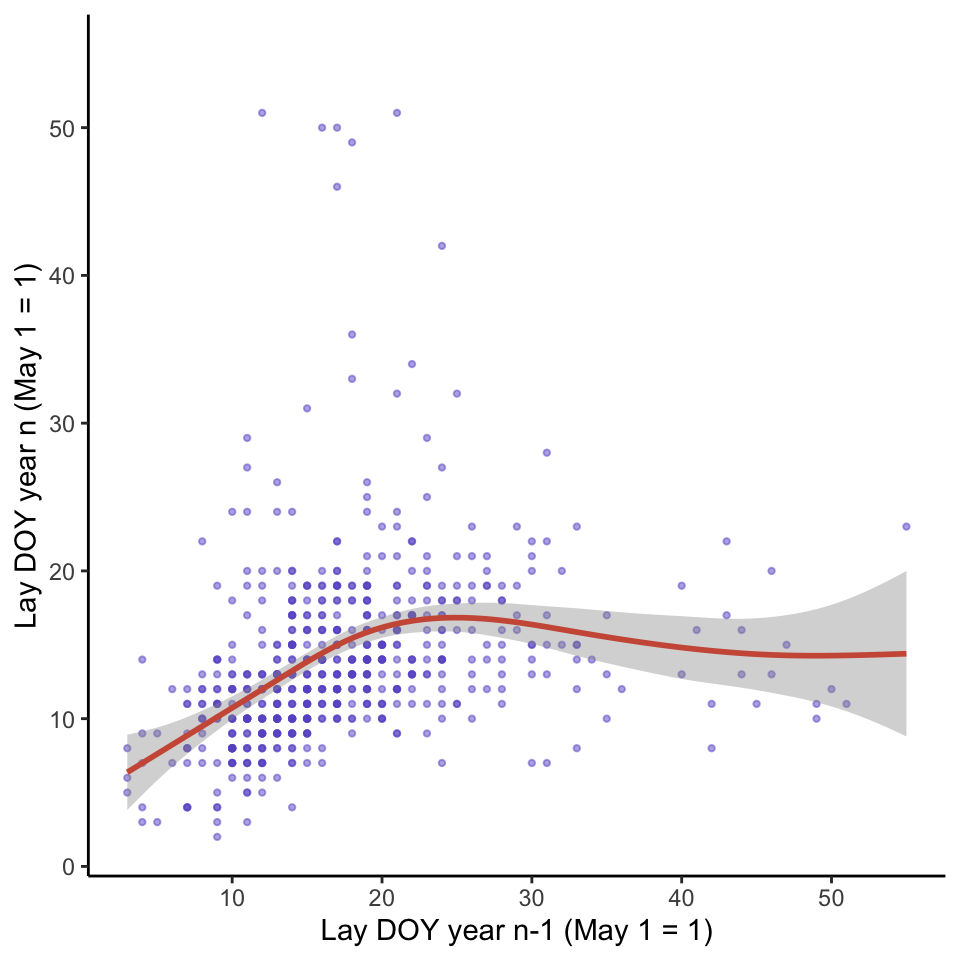So there really is a pretty strong correlation at least through those first ~25 days of the egg laying period. The Ecology paper includes those fans of outliers to the bottom right and upper left, but I actually wonder if it might be better to remove them (though it isn’t clear how to do that in an objective way). I suspect a lot of those are cases where a very early abandonment was not detected in one year or the other. I’m fitting the smoothing line here as a GAM rather than a linear model so it isn’t dragged down as much by those outliers. In any case, it’s a good start for asking how much lay dates change over-and-above what is expected from the individual identity based on temperature experienced.

I’m adding in several different weather metrics here for first year of each pair of observations. We could also add in weather in the second year, but I think for now it’s easier to try to capture that variation with a random effect of year. I also don’t have exact hatch or fledge dates in here because they weren’t stored in the Ecology paper, so I’m just using the estimated ranges. For a final analysis we should add those back in from the database and FoxPro. This code chunk is just looping through and adding in weather summarized in each of the time periods:

• Laying
• Incubation
• Day 0 - 8 after hatching
• Day 8 - 15 after hatching

And in these two ways:

• Average daytime temperature in that period (6am to 8pm)
• Number of days in the period where day time high is < 18.5 C
``````dw <- read.delim(here::here("1_raw_data/Input_Weather_Data.txt"))
dw <- subset(dw, dw\$station == "Airport")

d2 <- subset(d, is.na(d\$prev_lay) == FALSE)

for(i in 1:nrow(d2)){
sub <- subset(dw, dw\$year == d2\$current_year[i] - 1)
sub_lay <- subset(sub, sub\$doy > d2\$prev_lay[i] + 120 - 1 & sub\$doy < d2\$prev_lay[i] + d2\$clutch[i] + 1 + 120 & sub\$hour > 6 & sub\$hour < 20)
sub_inc <- subset(sub, sub\$doy > d2\$prev_lay[i] + 120 + d2\$clutch[i] + 1 & sub\$doy < d2\$prev_lay[i] + d2\$clutch[i] + 15 + 120 & sub\$hour > 6 & sub\$hour < 20)
sub_d8 <- subset(sub, sub\$doy > d2\$prev_lay[i] + 120 + d2\$clutch[i] + 15 & sub\$doy < d2\$prev_lay[i] + d2\$clutch[i] + 24 + 120 & sub\$hour > 6 & sub\$hour < 20)
sub_d15 <- subset(sub, sub\$doy > d2\$prev_lay[i] + 120 + d2\$clutch[i] + 24 & sub\$doy < d2\$prev_lay[i] + d2\$clutch[i] + 33 + 120 & sub\$hour > 6 & sub\$hour < 20)

lay_his <- as.data.frame(unique(sub_lay\$doy))
inc_his <- as.data.frame(unique(sub_inc\$doy))
d8_his <- as.data.frame(unique(sub_d8\$doy))
d15_his <- as.data.frame(unique(sub_d15\$doy))

for(k in 1:nrow(lay_his)){
lay_his\$day_high[k] <- max(na.omit(subset(sub_lay\$avg_temp_C, sub_lay\$doy == lay_his[k, 1])))
}
for(k in 1:nrow(inc_his)){
inc_his\$day_high[k] <- max(na.omit(subset(sub_inc\$avg_temp_C, sub_inc\$doy == inc_his[k, 1])))
}
for(k in 1:nrow(d8_his)){
d8_his\$day_high[k] <- max(na.omit(subset(sub_d8\$avg_temp_C, sub_d8\$doy == d8_his[k, 1])))
}
for(k in 1:nrow(d15_his)){
d15_his\$day_high[k] <- max(na.omit(subset(sub_d15\$avg_temp_C, sub_d15\$doy == d15_his[k, 1])))
}

d2\$avg_lay[i] <- mean(na.omit(sub_lay\$avg_temp_C))
d2\$cs_lay[i] <- nrow(subset(lay_his, lay_his\$day_high < 18.5))

d2\$avg_inc[i] <- mean(na.omit(sub_inc\$avg_temp_C))
d2\$cs_inc[i] <- nrow(subset(inc_his, inc_his\$day_high < 18.5))

d2\$avg_d8[i] <- mean(na.omit(sub_d8\$avg_temp_C))
d2\$cs_d8[i] <- nrow(subset(d8_his, d8_his\$day_high < 18.5))

d2\$avg_d15[i] <- mean(na.omit(sub_d15\$avg_temp_C))
d2\$cs_d15[i] <- nrow(subset(d15_his, d15_his\$day_high < 18.5))
}``````

# Distribution of temperature

Just to get an idea of how much variation there is, I’m plotting histograms for the average temperature and number of ‘cold snap’ days in each of the periods defined above. Here is the distribution for average daytime temperatures:

``````ggplot(data = d2, mapping = aes(x = avg_lay)) + geom_histogram(fill = "goldenrod") + theme_classic() + xlab("Avg. Laying")
ggplot(data = d2, mapping = aes(x = avg_inc)) + geom_histogram(fill = "goldenrod") + theme_classic() + xlab("Avg. Incubation")
ggplot(data = d2, mapping = aes(x = avg_d8)) + geom_histogram(fill = "goldenrod") + theme_classic() + xlab("Avg. Day 0-8")
ggplot(data = d2, mapping = aes(x = avg_d15)) + geom_histogram(fill = "goldenrod") + theme_classic() + xlab("Avg. Day 8-15")``````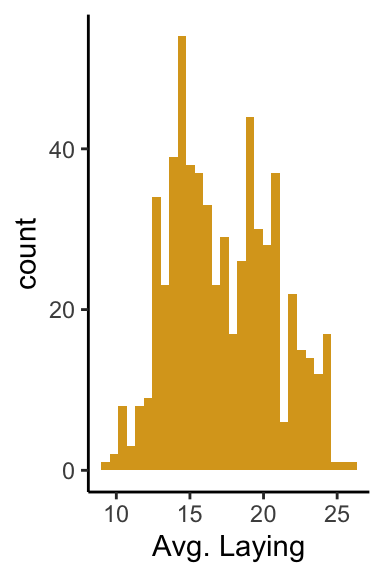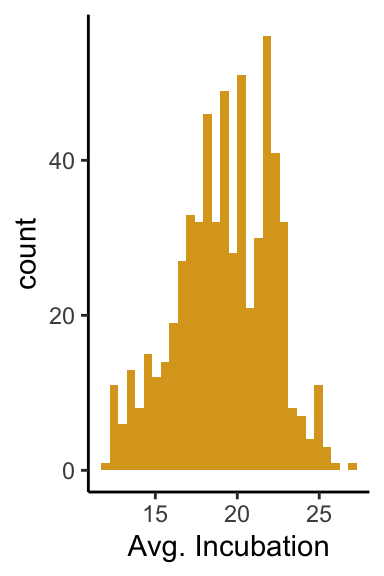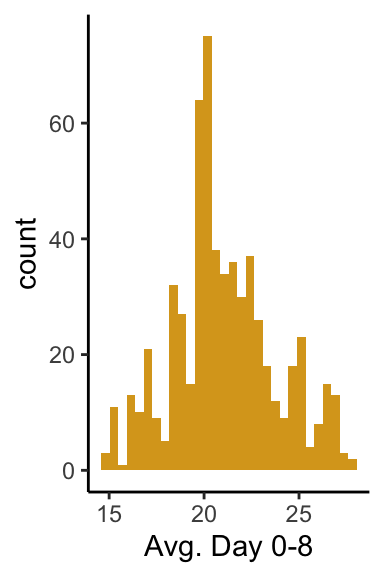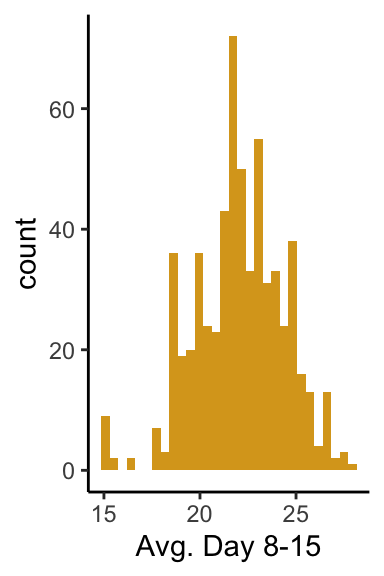And for the number of ‘cold snap’ days:

``````ggplot(data = d2, mapping = aes(x = cs_lay)) + geom_histogram(fill = "goldenrod", bins = length(unique(d2\$cs_lay))) + theme_classic() + xlab("CS Days Laying")
ggplot(data = d2, mapping = aes(x = cs_inc)) + geom_histogram(fill = "goldenrod", bins = length(unique(d2\$cs_inc))) + theme_classic() + xlab("CS Days Incubation")
ggplot(data = d2, mapping = aes(x = cs_d8)) + geom_histogram(fill = "goldenrod", bins = length(unique(d2\$cs_d8))) + theme_classic() + xlab("CS Days 0-8")
ggplot(data = d2, mapping = aes(x = cs_d15)) + geom_histogram(fill = "goldenrod", bins = length(unique(d2\$cs_d15))) + theme_classic() + xlab("CS Days 8-15")``````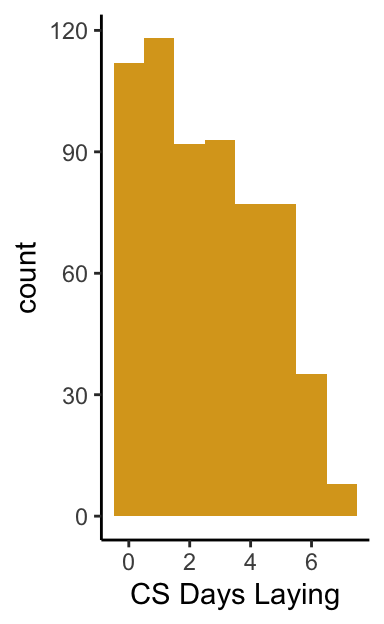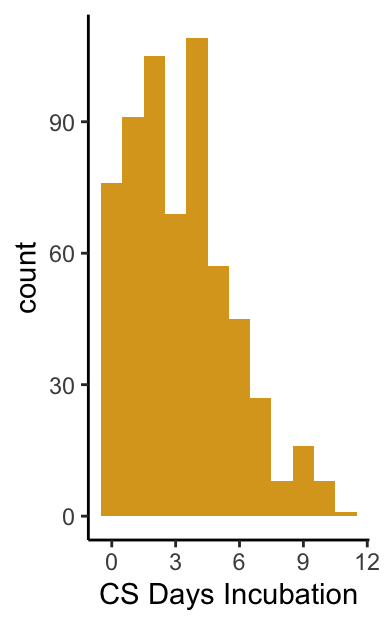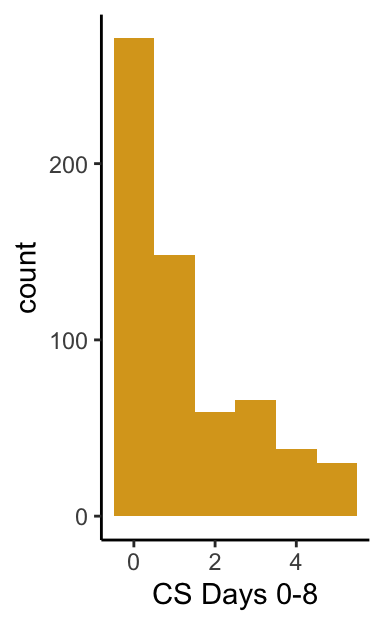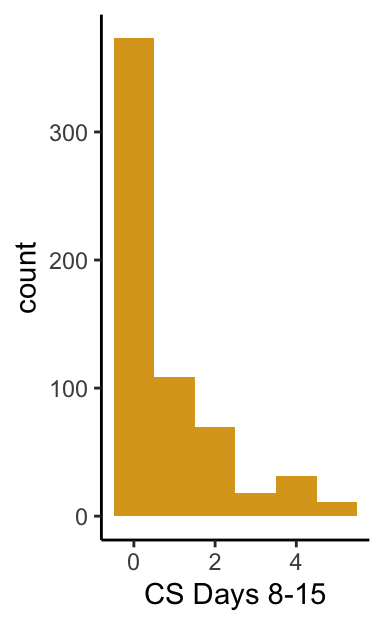So there is a fair amount of variation in temperature experienced during these periods and in the number of cold snap days, which isn’t surprising.

# Temperature vs. future laying

OK, now we’ve gotten around to being able to plot relationships between temperature and the time of laying in the next year. First I’m plotting this just for the raw values and then I’ll plot values that take into account change in laying date relative to each bird’s own expectation. First plotting for average temperatures then for number of cold snap days.

``````d2\$delta_lay <- d2\$doy_lay - d2\$prev_lay

ggplot(data = d2, mapping = aes(x = avg_lay, y = delta_lay)) + geom_point(col = "slateblue", size = 0.7, alpha = 0.5) +
geom_smooth(method = "lm", col = "coral3") + theme_classic() + xlab("Avg n-1 C Laying") + ylab("Delta Lay Date")
ggplot(data = d2, mapping = aes(x = avg_inc, y = delta_lay)) + geom_point(col = "slateblue", size = 0.7, alpha = 0.5) +
geom_smooth(method = "lm", col = "coral3") + theme_classic() + xlab("Avg n-1 C Incubation") + ylab("Delta Lay Date")
ggplot(data = d2, mapping = aes(x = avg_d8, y = delta_lay)) + geom_point(col = "slateblue", size = 0.7, alpha = 0.5) +
geom_smooth(method = "lm", col = "coral3") + theme_classic() + xlab("Avg n-1 C Day 0-8") + ylab("Delta Lay Date")
ggplot(data = d2, mapping = aes(x = avg_d15, y = delta_lay)) + geom_point(col = "slateblue", size = 0.7, alpha = 0.5) +
geom_smooth(method = "lm", col = "coral3") + theme_classic() + xlab("Avg n-1 C Day 8-15") + ylab("Delta Lay Date")

ggplot(data = d2, mapping = aes(x = cs_lay, y = delta_lay)) + geom_point(col = "slateblue", size = 0.7, alpha = 0.5) +
geom_smooth(method = "lm", col = "coral3") + theme_classic() + xlab("Cold Days Laying") + ylab("Delta Lay Date")
ggplot(data = d2, mapping = aes(x = cs_inc, y = delta_lay)) + geom_point(col = "slateblue", size = 0.7, alpha = 0.5) +
geom_smooth(method = "lm", col = "coral3") + theme_classic() + xlab("Cold Days Incubation") + ylab("Delta Lay Date")
ggplot(data = d2, mapping = aes(x = cs_d8, y = delta_lay)) + geom_point(col = "slateblue", size = 0.7, alpha = 0.5) +
geom_smooth(method = "lm", col = "coral3") + theme_classic() + xlab("Cold Days 0-8") + ylab("Delta Lay Date")
ggplot(data = d2, mapping = aes(x = cs_d15, y = delta_lay)) + geom_point(col = "slateblue", size = 0.7, alpha = 0.5) +
geom_smooth(method = "lm", col = "coral3") + theme_classic() + xlab("Cold Days 8-15") + ylab("Delta Lay Date")``````